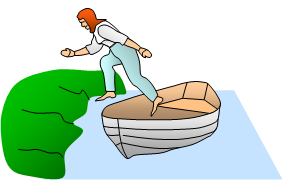# Conservation of momentumIf you are in a small boat and make a jump for the bank why does the boat move back the other way, dropping you in the water?

This is to do with the law of conservation of momentum.

This says that:-

Momentum is conserved in a collision or explosion in an isolated system where no external forces act. In other words the momentum before the collision or explosion is the same as that after it. This is true for ALL collisions and explosions

Before the jump the combined momentum of you and the boat was zero. Now since momentum must be conserved the combined momentum after the jump must also be zero. If you have a momentum in one direction the boat must have an equal momentum but in the opposite direction. If your mass is say a tenth of the boat's, the boat will move the other way at one tenth of your velocity.
(Remember that momentum is a vector)

Momentum before interaction = Momentum after interaction = 0 = MV+mv

where M and V are the mass and velocity of the boat and m and v are the mass and velocity of the person.

So V = - mv/M, the minus sign meaning that the boat is moving in the opposite direction to the person.

In a collision the same law of conservation of momentum applies. If a mass m1 moving at a velocity u1 collides with a mass m2 moving at a velocity u2 such that after the collision m1 moves at v1 and m2 moves at v2 and so:-

momentum before collision = momentum after collision

m1u1 + m2u2 = m1v1 + m2v2

Example problems
If a mass of 3.5 kg is moving left to right at 5 ms-1 collides with a mass of 4.0 kg moving right to left at 3.0 ms-1 and they stick together find the final velocity of the combined masses.

Momentum before impact = 3.5x5.0 + [-4.0x3.0] = 5.5 Ns but this must equal the momentum after the collision i.e. total mass x final velocity
Notice that one of the velocities is negative showing that the ball was moving right to left.
Mass afterwards = 7.5 kg
Therefore velocity afterwards = 5.5/7.5 = 0.73 ms-1 this is positive showing that after collision the combined two balls move from left to right.

A VERSION IN WORD IS AVAILABLE ON THE SCHOOLPHYSICS USB